Next: Proudman Equations Up: Terrestrial Ocean Tides Previous: Auxilliary Eigenfunctions

# Gyroscopic Coefficients

Let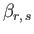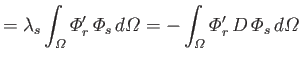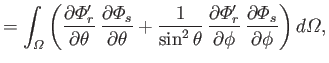(12.269)

where use has been made of Equations (12.235) and (12.236). It follows from Equations (12.262) and (12.263) that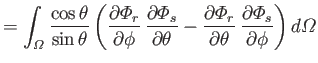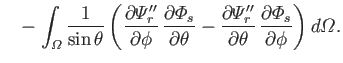(12.270)

However, the second term on the right-hand side of the previous equation integrates to zero with the aid of Equation (12.261). Hence, we are left with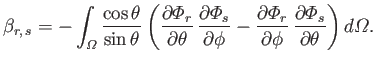(12.271)

Let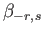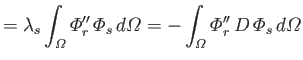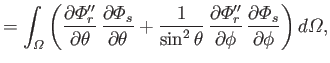(12.272)

where use has been made of Equations (12.235) and (12.236). It follows from Equations (12.272) and (12.273) that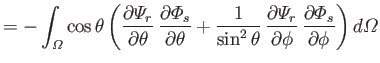(12.273)

However, the second term on the right-hand side of the previous equation integrates to zero with the aid of Equation (12.271). Hence, we are left with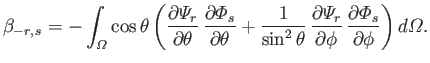(12.274)

Let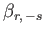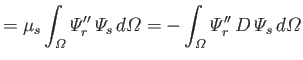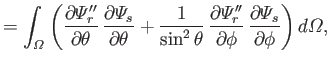(12.275)

where use has been made of Equations (12.248) and (12.261). It follows from Equations (12.262) and (12.263) that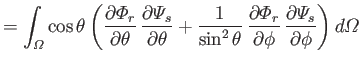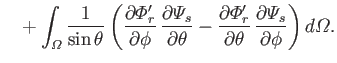(12.276)

However, the second term on the right-hand side of the previous equation integrates to zero with the aid of Equation (12.249). Hence, we are left with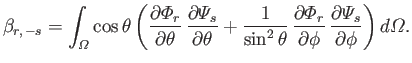(12.277)

Finally, let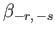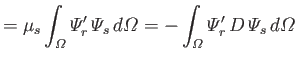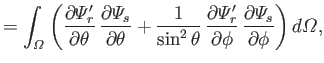(12.278)

where use has been made of Equations (12.248) and (12.271). It follows from Equations (12.272) and (12.273) that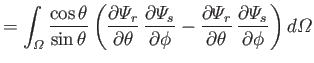(12.279)

However, the second term on the right-hand side of the previous equation integrates to zero with the aid of Equation (12.249). Hence, we are left with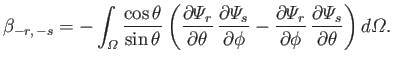(12.280)

Incidentally, the,,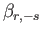, andare known collectively as gyroscopic coefficients (Proudman 1916).Next: Proudman Equations Up: Terrestrial Ocean Tides Previous: Auxilliary Eigenfunctions
Richard Fitzpatrick 2016-03-31